# HiSET Mathematics: Quadratic Equations Chapter Exam

Exam Instructions:

Choose your answers to the questions and click 'Next' to see the next set of questions. You can skip questions if you would like and come back to them later with the yellow "Go To First Skipped Question" button. When you have completed the practice exam, a green submit button will appear. Click it to see your results. Good luck!

### Page 1

#### Question 1 1. What is the b-value of the quadratic equation below?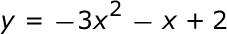#### Question 2 2. Find the roots of the equation.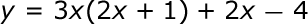#### Question 4 4. Rewrite the quadratic below in standard form: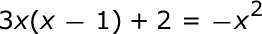#### Question 5 5. Find the solution to the equation below.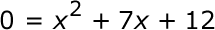### Page 2

#### Question 7 7. Find the solution to the equation below.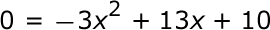#### Question 8 8. Find the roots of the equation below.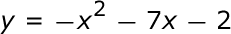### Page 3

#### Question 11 11. Solve the following equation.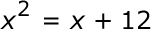#### Question 14 14. Use the quadratic formula to find the solutions to the equation below.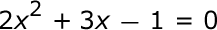### Page 4

#### Question 17 17. In order to complete the square on the following equation, what value would you add to both sides?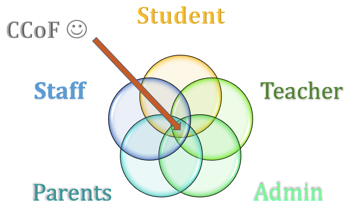#### Question 18 18. If the equation below told you a ball was 'h' feet in the air after 't' seconds, what would be the first step to finding out when it hit the ground?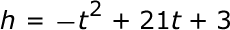### Page 5

#### Question 21 21. When completing the square for the quadratic equation shown below, how is c calculated?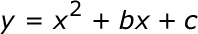#### Question 22 22. Which of the following are the solutions to the equation below?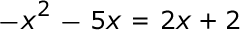#### HiSET Mathematics: Quadratic Equations Chapter Exam Instructions

Choose your answers to the questions and click 'Next' to see the next set of questions. You can skip questions if you would like and come back to them later with the yellow "Go To First Skipped Question" button. When you have completed the practice exam, a green submit button will appear. Click it to see your results. Good luck!

Support